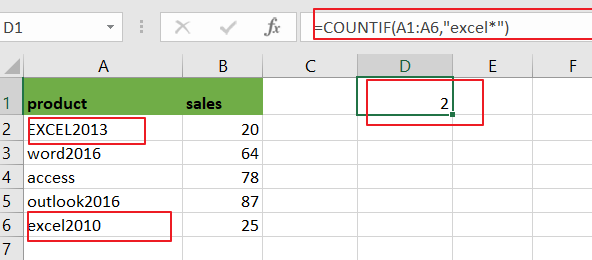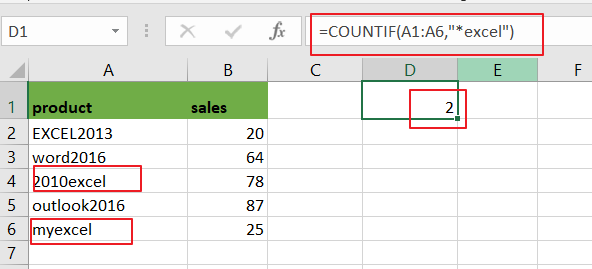# How to Count Cells That Begin with Specific Text in Excel

This post will guide you how to count cells that begin with a specific text string in a given range cells using a formula in Excel 2013/2016.How do I count cells the number of cells that begin with a certain text string in Excel.

## Count Cells that Begin with Specific Text

Assuming that you want to count the number of cells that have the certain letters(“excel”)from the word approved as the first five characters in a selected range(A1:A6).In this case, you can use a formula based on the COUNTIF function.

Enter the following formula in a blank cell, and press Enter key:

=COUNTIF(A1:A6,”excel*”)Note: A1:A6 is the data range that you want to use. “excel*” is a condition that that data need to be matched, and it means that the text that cells should begin with “excel”.”*” character is a wildcard that matching any number of characters.

Now Let’s see how this formula works:

The COUNTIF function can be used to count the number of cells in a selected range that begin with a certain pattern. And the asterisk character is a wildcard in Excel that indicates that matching any number of characters in cells. Then it returns a number that matching the given pattern.

If you want to counts the number of cells in a specified range of cells that end with a certain text(“excel”), and you can also use the CONNTIF function to create a similar formula with the pattern “*excel”as the criteria. The formula is like below:

=COUNTIF(A1:A6,”*excel”)### Related Functions

• Excel COUNTIF function
The Excel COUNTIF function will count the number of cells in a range that meet a given criteria. This function can be used to count the different kinds of cells with number, date, text values, blank, non-blanks, or containing specific characters.etc.= COUNTIF (range, criteria)…